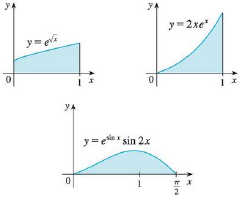Chapter 5.5, Problem 79E### Single Variable Calculus: Early Tr...

8th Edition
James Stewart
ISBN: 9781305270343

#### Solutions

Chapter
Section### Single Variable Calculus: Early Tr...

8th Edition
James Stewart
ISBN: 9781305270343
Textbook Problem

# Which of the following areas are equal? Why?To determine

To find: The equal are of the equation from the following equation:

y=ex, y=2xex, and y=esinxsin2x

Explanation

Given:

The equations are

y=ex,(0,1).

y=2xex,(0,1)

y=esinxsin2x,(0,π2)

Calculation:

Express the area of function by using formula:

A=abydx (1)

The equation is,

y=ex

Calculate the area of the equation:

Substitute 0 for a, 1 for b, and ex for y in Equation (1).

A1=01exdx (2)

Consider u=x (3)

Differentiate both sides of the Equation (3).

du=12xdx2xdu=dx (4)

Substitute u for x in Equation (4).

2udu=dx

Calculate the lower limit value of u using Equation (3).

Substitute 0 for x in Equation (3).

u=0=0

Calculate the upper limit value of u using Equation (3).

Substitute 1 for x in Equation (3).

u=1=1

Apply lower and upper limits for u in Equation (2).

Substitute u for (x) and (2udu) for dx in Equation (2).

A1=01eu(2udu)=201euudu (5)

Integrate Equation (5).

A1=2[ueu1.eu]01=2[ueueu]01=2[(1×e1e1)(0×e0e0)]

=2(0+1)=2

The equation is

y=2xex

Calculate the area of the equation:

Substitute 0 for a, 1 for b, and 2xex for y in Equation (1).

A2=012xexdx (6)

Integrate Equation (6)

### Still sussing out bartleby?

Check out a sample textbook solution.

See a sample solution

#### The Solution to Your Study Problems

Bartleby provides explanations to thousands of textbook problems written by our experts, many with advanced degrees!

Get Started

#### Expand each expression in Exercises 122. (2xy)y

Finite Mathematics and Applied Calculus (MindTap Course List)

#### In Exercises 2336, find the domain of the function. 29. f(x)=5x

Applied Calculus for the Managerial, Life, and Social Sciences: A Brief Approach

#### For , f(x) = 0 2 3 f(3) does not exist

Study Guide for Stewart's Multivariable Calculus, 8th